# IBPS Clerk Mains Reasoning (Day-20)

Dear Aspirants, Our IBPS Guide team is providing new series of Reasoning Questions for IBPS Clerk Mains 2021 so the aspirants can practice it on a daily basis. These questions are framed by our skilled experts after understanding your needs thoroughly. Aspirants can practice these new series questions daily to familiarize with the exact exam pattern and make your preparation effective.

Start Quiz

Matrix based puzzle

Directions (1-4): Study the following information carefully and answer the below questions.

There is a 3*5 matrix that can produce signals which in turn help in the illumination of some bulbs. The row of the matrix is denoted by @, % and # from the bottom to top respectively and the columns are denoted by A, B, C, D and E from left to right respectively.

@ row contains a number which are consecutive multiples of 13, starting from 39 from left to right. % row contains a number which are consecutive multiples of 15, starting from 30 from left to right. # row contains a number which are consecutive multiples of 17 starting from 34 from right to left.

The matrix helps in producing signals which can be either a single string of number X or two line string X and Y. There are four lights P, Q, R and S. Based on the outcome of the string mentioned above one of the light blinks.

a) If the outcome is below 50 then P will blink

b) If the outcome range is 51-200, then Q will blink

c) If the outcome range is 201-270, then R will blink

d) If the outcome is greater than 270 then S will blink

For outcome of the string:

1) If the string has all even numbers then the outcome of the string is obtained by adding all the numbers.

2) If an odd number is followed by an even number then, the one’s place of all the two digit numbers is deleted and 10th place is multiplied to get the outcome.

3) If the string has a prime number, then the 10th place is deleted from each of the two digit numbers and the remaining numbers are multiplied.

4) If no above logic is followed then outcome is an addition of the number.

1) Which of the following bulb will illuminate for the given input

X = @B%A#[email protected]?

E. Cannot be determined

2) Which of the following bulb will illuminate for the given input

X = %[email protected]#E%A?

E. Cannot be determined

3) Which of the following bulb will illuminate for the given input

X = @B#E%E#A?

E. Cannot be determined

4) Which of the following bulb will illuminate for the given input

X = @E#[email protected]%B?

E.Cannot be determined

Number series conditions based

Direction (5-7): Two rows of numbers are given. The resultant number in each row is to be worked out separately based on the following rules and the question below the rows of numbers is to be answered. The operations of numbers progress from left to right.

Step I: If an odd number is followed by an even number then the resultant will be the addition of both the numbers.

Step II: If an even number is followed by a perfect square then the resultant will be the subtraction of the square number from the even number.

Step III: If an odd number is followed by another odd number then the resultant will be the addition of both the numbers.

Step IV: If an even number is followed by even number then the resultant comes by multiplying the number.

Note: If two conditions apply for the same number then the step which comes first as in ascending order is only considered. (If 48 and 16 ->  Step II and Step IV followed for this number so, we can take only step II)

5) Find the sum of the resultants of the two rows.

21        5          9

8          6          16

A.30

B.33

C.49

D.16

E.None of these

6) Find the sum of the resultants of the two rows, If X is the resultant of the second row.

X         25        2

3          7          6

A.32

B.57

C.48

D.40

E.97

7) Find the difference between the resultant of the first and the second row.

16        2          25

5          8          9

A.17

B.39

C.20

D.15

E.None of these

Direction sense

Directions (8-10): Study the following information carefully and answer the below questions.

Three friends Roy, Tom and Mike start walking towards town hall from their respective home. Point B is 28m south of point M. Tom standing at point A, walks 12m towards east to reach point E, takes a left turn of 24m to reach point L. Point G is 48m south of point J and is 28m east of point L. From point J, Tom takes a left turn of 16m to reach point H, which is 32m south of point C. Roy standing at point M, which is 28m west of point I. Point D is 12m south of point F and is 16m east of point B. Mike standing at point P, walks 28m towards west to reach point N. He again takes a left turn of 36m to reach point Q, which is 18m east of town hall. Point K is 12m west of point C and is 48m north of town hall. Point F is 42m west of point Q.

8) What is the position of point L with respect to the town hall?

A.South-East

B.South

C.North- East

D.West

E.None of the above

9) What is the shortest distance between I and town hall?

A.18√2m

B.14m

C.20m

D.16√3m

E.None of the above

10) Which of the following statement is not true?

A.Point D is south-west of point Q.

B.Point C is north-west of point J.

C.Point F is west of point G.

D.Roy is north of point F.

E.More than one statements are not true.

Directions (1-4) :

From the given data we can create the following table;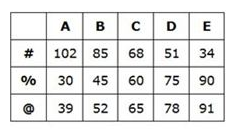X = @B%A#[email protected]

X = 52 30 102 78

Here all the outcome of the string is in even number; So,

X = 52+30+102+78=262

X = %[email protected]#E%A

X = 45 65 34 30

Here in the outcome of the string odd number is followed by an even number; So,

X = 4 x 6 x 3 x 3 = 216

X = @B#E%E#A

X = 52 34 90 102

Here all the outcome of the string is in even number; So,

X = 52+34+90+102=278

X = @E#[email protected]%B

X = 91 51 39 45

Here in the outcome of the string there is a prime number; So,

X = 1 x 1 x 9 x 5 = 45

Directions (5-7) :

1st row step III applied 21+5=26; Step II applied 26-9=17

2nd row step IV applied 8×6=48; step II => 48-16=32

Sum of resultant is 32+17=49

2nd row step III 3+7=10 step IV => 10×6=60

1st row Step II 60-25=35; Step I => 35+2=37

Sum of two rows are 60+37 =97

1st row step IV => 16×2=32, step II => 32-25=7

2nd row step I => 5+8=13, step III => 13+9=22

Difference is 22-7=15

Directions (8-10) :

We have:

• Mike standing at point P, walks 28m towards west to reach point N.
• Point N is 36m north of point Q.
• Point Q is 18m east of town hall.
• Point F is 42m west of point Q, that means point F must be 24m west of town hall.

Based ontheabove given information we have: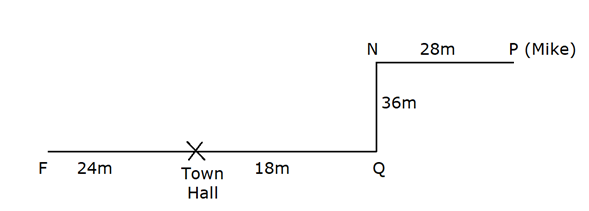Again, we have:

• Point D is 12m south of point F and is 16m east of point B, that means point F is 12m north of point D.
• From point M Roy walks 28m towards his left to reach point B.
• Point B is 28m south of point M.
• Roy standing at point M, which is 28m west of pointI.

Based on the above given information we have: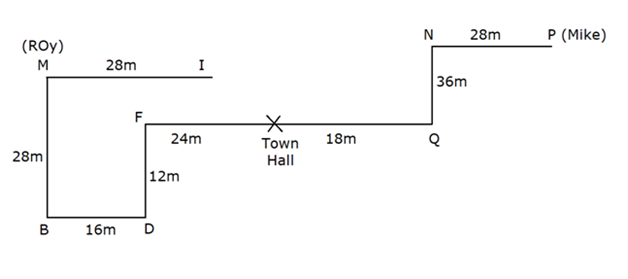Again, we have:

• Point K is 12m west of point C and is 48m north of town hall, that means point K is 48m north of town hall.
• Point H is 32m south of point C.
• From point J, Tom takes a left turn of 16m to reach point H.
• Point G is 48m south of point J and is 28m east of point L, that means point J is 16m east of point H.
• Tom standing at point A, walks 12m towards east to reach point E, takes a left turn of 24m to reach point L, which means point L is 24m north of point E.

Based on the above given information we have thefinal arrangement as follow: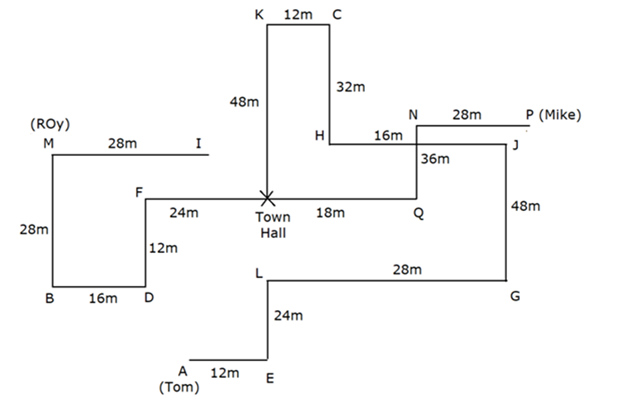We have: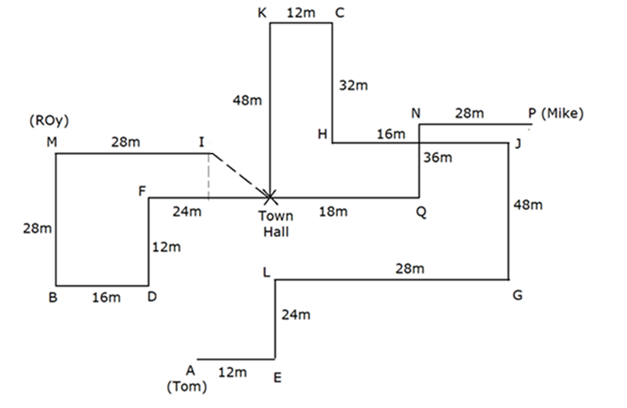Clearly, Base = (24 + 16) – 28 = 12m.

Perpendicular = (28 – 12) = 16m

Shortest distance = √(162 + 122) = 20m.